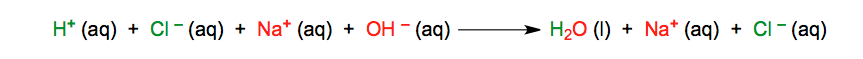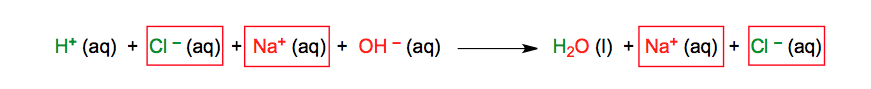Clutch Prep is now a part of Pearson
Ch.4 - Chemical Quantities & Aqueous ReactionsWorksheetSee all chapters

# Net Ionic Equation (IGNORE)

See all sections
Sections
Solutions
Molarity
Osmolarity
Dilutions
Solubility Rules
Electrolytes
Molecular Equations
Gas Evolution Equations
Solution Stoichiometry
Complete Ionic Equations
Calculate Oxidation Numbers
Redox Reactions
Balancing Redox Reactions: Acidic Solutions
Balancing Redox Reactions: Basic Solutions
Activity SeriesJules Bruno

A molecular equation is represented by a balanced chemical equation where the reactants and products are written in their neutral forms. By eliminating its spectator ions the molecular equation is transformed into the net ionic equation

How to determine the Net Ionic Equation

A molecular equation can only result if you produce a solid, liquid or gas as a product. To determine if these states of matter are formed you use the solubility rules and your understanding of weak and strong electrolytes

Take for instance the acid-base reaction between hydrochloric acid, HCl, and sodium hydroxide, NaOH.

STEP 1: Write the molecular equation and determine the phases of your products.Acid-Base-Molecular-Equation

In this example the chemical reaction occurs because a liquid was formed. Chemical reactions that produce a solid as a product are termed precipitation reactions.

STEP 2: Based on your understanding of soluble ionic compounds and electrolytes we must give the total or complete ionic equation. Keep in mind that if the coefficients of the compounds were different they would change the number of each ion produced.Total or Complete Ionic Equation

In this step we break up only the soluble aqueous compounds into ions, while solids, liquids and gases stay intact.

STEP 3: The spectator ions represent the ions that remain unchanged in a chemical reaction. They look the same on both sides of the chemical equation.Total or Complete Ionic Equation (Spectator Ions)

The spectator ions represent the ions that haven’t reacted and remain suspended and dissolved in the solution.Eliminating Spectator Ions

Eliminating the spectator ions gives the net ionic equation.Net Ionic EquationJules Bruno

Jules felt a void in his life after his English degree from Duke, so he started tutoring in 2007 and got a B.S. in Chemistry from FIU. He’s exceptionally skilled at making concepts dead simple and helping students in covalent bonds of knowledge.